#### How to Calculate Median in Excel

In this article, you’ll learn how to use the median function. It can be used to calculate the median of given numbers in Excel.

The median is just the middle of a set of numbers. But if there is no mean value in the set, the median is the average of the two mean values.

• MEDIAN of (1, 2, 3, 4, 5) is equal to 3
• MEDIAN of (1, 2, 3, 4, 5, 6) equals 3.5, which is an average of 3 and 4

## How does the MEDIAN function work?

The MEDIAN function works by first sorting the numbers in the range in ascending order. If the number of numbers is odd, the MEDIAN function returns the middle number. If the number of numbers is even, the MEDIAN function returns the average of the two middle numbers.

## Syntax of Median function

The syntax for the MEDIAN function is:

=MEDIAN(number1, [number2, …])

Number 1: This is required.

Number 2 – 255: This is optional, going all the way to 255, for which the user wants the median of.

You can use up to 255 numbers in your MEDIAN formula.

## Examples of Median function

Here are some examples of how to use the MEDIAN function in Excel:

### Median of an odd number of values

In case of odd number of values, median is just a middle value.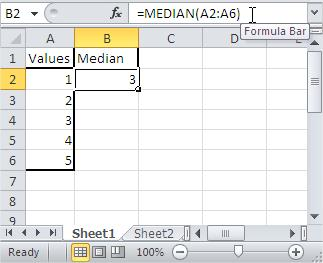### Median of an even number of values

For an even number of values, the median is the mean of the two middle values. Here it is average of 3 and 4, which is 3.5.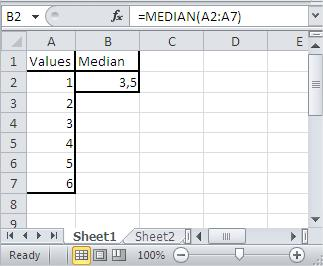### Median of selected cells

You can type addresses of cells for which you want to calculate median in Excel.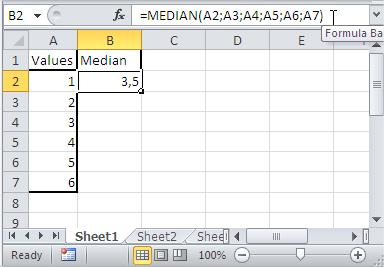### Median of typed numbers

Instead of addresses you can also use number. Using this method you don’t need any table of data. Notice that formula is not connected with column A in the picture.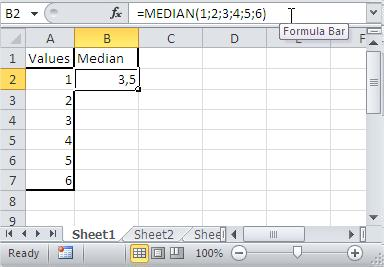### Median – mix of above examples

You can also use all above methods together.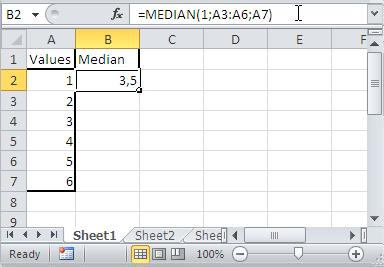## Tips for using the MEDIAN function in Excel

The MEDIAN function is a non-volatile function, which means that it does not recalculate its value whenever the workbook is recalculated. This can be useful if you want to keep the median of the numbers up to date, but it can also be a problem if you are using the MEDIAN function in a formula that should not be recalculated every time the workbook is opened.

The MEDIAN function can be used to calculate the median of any range of numbers, regardless of their size or shape.

The MEDIAN function can be used in conjunction with other functions to perform more complex calculations. For example, you could use the MEDIAN function to calculate the interquartile range of a set of data.